Scripting

# How to Calculate the Square Root in Python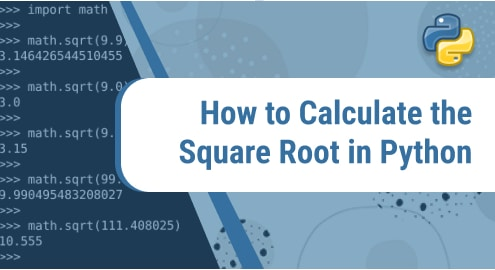Python provides us with the built-in function of the square root which is a part of the math module. To find the square root of a number, we import the math module first then we can use the square root function.

## Square root function:

This function takes one argument and returns the square root which is a float point number. The argument for the square root function should be a positive integer.

### Syntax:

The syntax for the square root function is as follows:

`math. sqrt(n)`

Firstly, we write the module name “math” then use dot notation (.) and then write function name “sqrt” with one argument enclosed in round brackets. Let’s understand this by a few examples.

### Example 1:

```import math

print("The square root of 36 is:",math.sqrt(36))```

Output:

The square root of 36 is: 6.0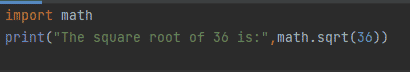Output: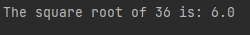In example 1, we imported the math library by using the import keyword. As we can see in Figure 1 we calculated the square root of 36. To display the result we used a print statement. The result is 6.0 a floating-point in figure2.

### Example 2:

```import math

n=eval(input("Enter a number to calculate square root"))

print("The square root of number is:",math.sqrt(n))```

Output:

Enter a number to calculate square root 45

The square root of the number is: 6.708203932499369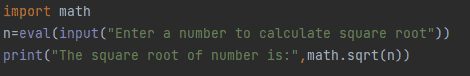Output: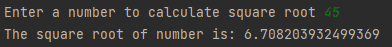We can make the function dynamic by asking the user to enter a number of their choice. In Figure 3 we used the eval function to convert input into a number. A message will appear on the screen as shown in Figure 4. After entering the desired number we’ll get the result.

### Example 3:

```from math import sqrt

def pythagoras(x, y):

if x<= 0 or y <= 0:

return

return sqrt(x * x + y * y)

print(pythagoras(7, 4))```

Output:

8.06225774829855Output: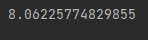In example 3, we have just imported only the sqrt function from the math module. In Figure 5, we defined a function Pythagoras to calculate the square root of the sum of the square of its two parameters. If the condition has been used to check that both numbers should be greater than zero. If this condition is true then the square root will be calculated. If any parameter is less than zero then the function will exit as the return keyword is used here. In Figure 5 we have called a function with two positive integer arguments. So we get the output of a floating integer as shown in Figure 6.

### Example 4:

```from math import sqrt

def pythagoras(x, y):

if x<= 0 or y <= 0:

return

return sqrt(x * x + y * y)

print(pythagoras(-7, 4))```

Output:

None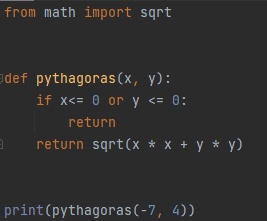Output: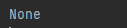In Figure 7, we are calling the same Pythagoras function using a negative argument. The variable x value is -7 and the variable y value is 4. So as the function will be called it will check if the condition is true or not. Here in example 4, if the condition is true so first return statement will be executed. As we cannot find the square root of negative integers, so the function will output none.